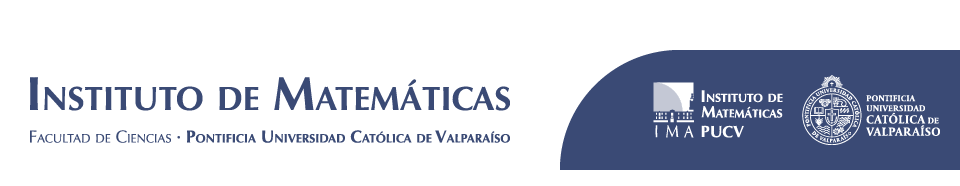﻿ Proyecto Fondecyt Iniciación “Cubic Periodic Curves and rescaling limits” | Instituto de Matemáticas# Proyecto Fondecyt Iniciación “Cubic Periodic Curves and rescaling limits”

## Resumen

We focus on describing the parameter spaces of cubic complex polynomials and complex quadratic rational maps with respect to their behavior under iteration on the Riemann sphere. Both of these spaces are interesting as they are a natural ground to generalize the results already known for quadratic polynomials and they present enough differences to force to develop tools that will be necessary to understand holomorphic dynamics of polynomials and rational maps of any degree.

Cubic polynomials

The space of cubic polynomials of the form
P_{a,b}(z):=z^3-3a^2z+b,
where (a,b) in C^2 is identified to C^2. To understand the organization of this space with respect to the dynamical behavior of its elements, we consider the subspace S_n of polynomials P_{a,b} for which the critical point +a has exact period n under iteration by P_{a,b}. We are interested in describing the dynamical structure of this curve in the spirit of previous works of John Milnor. For that purpose we will to work on two directions.

We will give a constructive proof of the following result:
Theorem [A.-Kiwi]: The curves S_n are irreducible algebraic curves for all n.

We will further the work already accomplished to proove the following conjecture
Conjecture: Let Q be any quadratic polynomial with a period n critical point. The connectedness locus Csubset S_n contains a homeomorphic copy of the filled Julia set of Q cut and opened along its Hubbard tree.

We consider the locus V_n in the moduli space of rational maps where the elements have a specific critical point has exact period \$ninN\$. We are interested in the following conjecture.

Conjecture:
The algebraic set V_n is irreducible for all n.

We propose to move a step forward in the determination of this conjecture by studying the compactification of the moduli space of rational maps. More precisely we are interested in the phenomena of rescaling limits. Relying on the approaches of Jan Kiwi and myself, we will study the realizability of dynamical systems on trees encoding rescaling limits as limits of rational maps.

Nombre del proyecto: Cubic Periodic Curves and rescaling limits

Código: 11170276

Fuente de financiamiento: Fondecyt

País: Chile

Fecha de inicio del proyecto: 01/11/2017

Fecha fin del proyecto: 31/10/2020

Compartir esta información en:

Compartir